# Looping Tutorial in C programming

C language looping tutorial: In this article, we will learn about the concept of loops in C programming language with definition, flow charts and Examples.
Submitted by Sneha Dujaniya, on July 04, 2018

## Loops in C language

Loops are used to repeat a part of the program specified number of times or until a specific condition is being specified.

There are total three ways in which we can achieve this:

1. The for loop
2. The while loop, and
3. The do...while loop

These loops are explained in detail as under.

### 1. The for loop

This is the most commonly used loop by the programmers and requires us to initialize three conditions in a single line at the start of the loop.

Syntax of 'for' loop:

```    for (initialize ; condition ; increment)
{
//body of the loop
//Code to be repeated till the test condition;
}
```

Flow chart: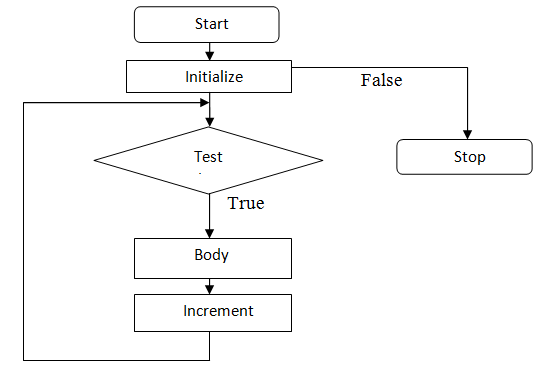Let us see how the loop functions?

Problem 1: Input two integers and find their average.

Solution: (Flow chart)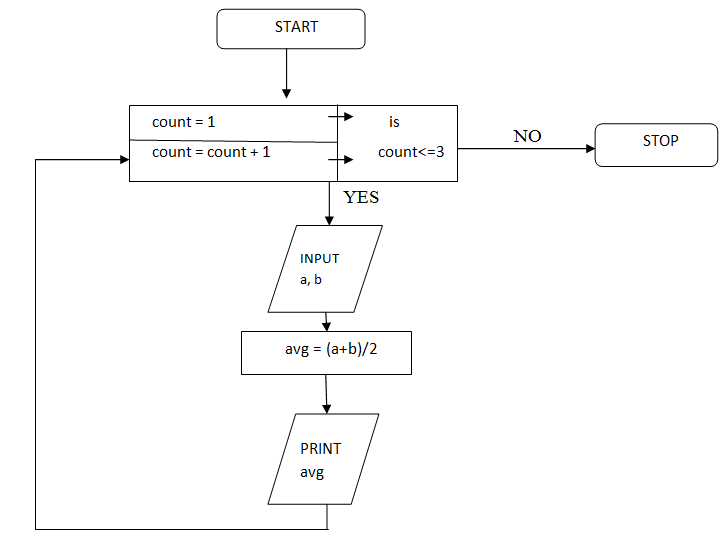C Language Code:

```#include <stdio.h>

int main()
{
int  a, b, avg, count ;

for(count = 1; count<=3; count++)
{
printf("Enter values of a and b: ");
scanf("%d %d",&a,&b);
avg=(a+b)/2;
printf("Average = %d" , avg);
}

return 0;
}
```

Output

```    Enter the value of a and b: 2 4
Average = 3
Enter the value of a and b: 2 3
Average = 2
Enter the value of a and b: 2 6
Average = 4
```

Explanation:

• When the loop begins for the first time, the value of count is set to be 1.
• Now, the condition count<=3 is tested. If the condition is true, the control enters into the loop and the body is executed once.
• After reaching the closing brace of the loop, the control is sent back to the for statement where the value of count is incremented by 1.
• After this, the condition count<=3 is again checked to see if the value of count is still within the range 1 to 3.
• If the condition stands true, the loop is executed again. This happens till the value of count becomes 3.
• When the value of count becomes 4, the loop terminates and the control is transferred to the statement after for (if any).

Now, it is not necessary that we always write the initial, test condition and increment in the same as stated above. There are many other ways to do so. Let us see some of them:

Problem 2: Print integers from 1 to 5.

C code:

```#include <stdio.h>

int main()
{
int i; //loop counter

//method 1
printf("Method 1...\n");
for(i=1; i<=5; i++)
printf("%d ",i);
printf("\n");

//method 2 (increment is in printf statement)
printf("Method 2...\n");
for(i=1; i<=5; )
printf("%d ",i++);
printf("\n");

//method 3 (printf statement with ++ in third section)
printf("Method 3...\n");
for(i=1; i<=5; printf("%d ",i++));
printf("\n");

//method 4
//initialization of loop before for statement
//increment is with the printf statement
printf("Method 4...\n");
i=1;
for( ; i<=5; )
printf("%d ",i++);

return 0;
}
```

Output

```    Method 1...
1 2 3 4 5
Method 2...
1 2 3 4 5
Method 3...
1 2 3 4 5
Method 4...
1 2 3 4 5
```

### 2. The while loop

This loop is mostly suitable for conditions where we have to perform a task a fixed number of times. For example, writing the data of 10 students of a class.

Syntax of 'while' loop

```    while (test_condition)
{
//body
//Code to be repeated till the test condition;
//other statement like ++/--
}
```

Flow chart: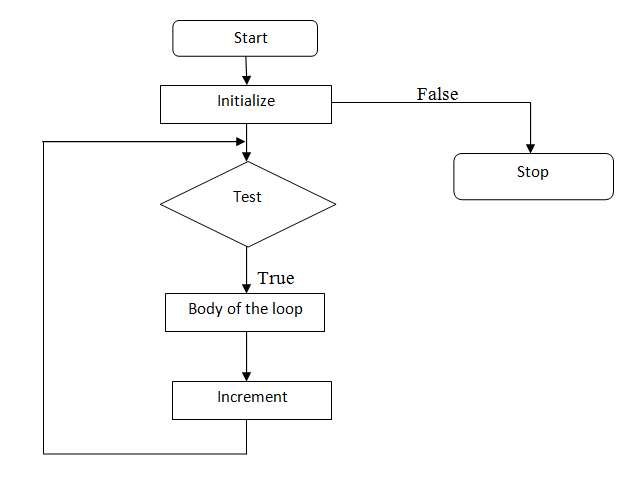Let us see how the loop functions?

Problem 1: Input two integers and find their average.

Solution: (Flow chart)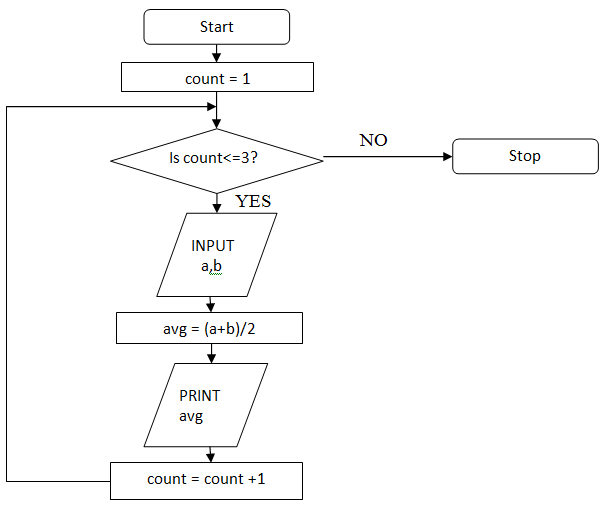C Language Code:

```#include <stdio.h>

int main()
{
int  a, b, avg, count ;

count =1;

while( count<=3 )
{
printf("Enter values of a and b: ");
scanf("%d %d",&a,&b);
avg=(a+b)/2;
printf("Average = %d" , avg);
}

return 0;
}
```

Output

```    Enter the value of a and b: 2 4
Average = 3
Enter the value of a and b: 2 3
Average = 2
Enter the value of a and b: 2 6
Average = 4
```

Explanation:

• Here, as long as the condition within the parenthesis stands true, all the statements inside the loop are executed.
• Which means that the while loop will be executed thrice.
• To be noted, if we do not write the condition in which we increment the value of count, the loop will become an infinite loop. This happens because the condition count<=3 stands true forever.
• Another important point is, there should be no semicolon after the while statement otherwise the loop will become indefinite.

Now, just as we saw in the for loop, there can be various ways in which we can achieve this result.

Problem 2: Print integers from 1 to 5.

C code:

```#include <stdio.h>

int main()
{
int i; //loop counter

//method 1
printf("Method 1...\n");
i=1;
while(i<=5)
{
printf("%d ",i);
i++;
}
printf("\n");

//method 2 (increment is in printf statement)
printf("Method 2...\n");
i=1;
while(i<=5)
{
printf("%d ",i++);
}
printf("\n");

return 0;
}
```

Output

```    Method 1...
1 2 3 4 5
Method 2...
1 2 3 4 5
```

### 3. The do...while loop

The do...while loop is almost the same as while loop except for one difference.

As an important point, the do...while loop is always executed at least once, unlike the other loops. This happens because the loop runs for the first time and then at the end, the condition is checked to be true or not. If it stands true, the loop runs again and if false, the control comes out.

Syntax of 'do...while' loop:

```    do
{
//body
//Code to be repeated till the test condition;
//other statement like ++/--
}while(test_condition);
```

Flow chart: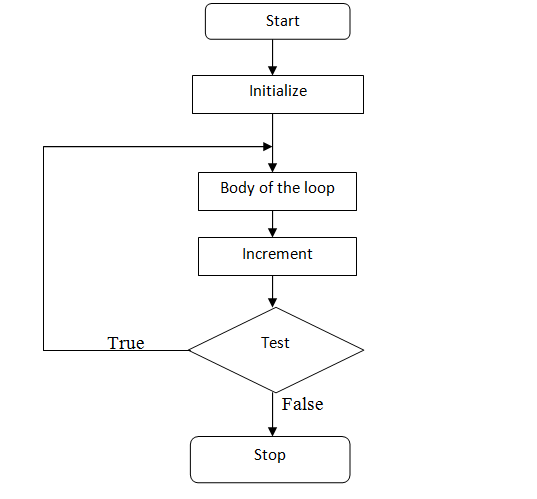Let us see how the loop functions?

Problem 1: Input two integers and find their average.

Solution: (Flow chart)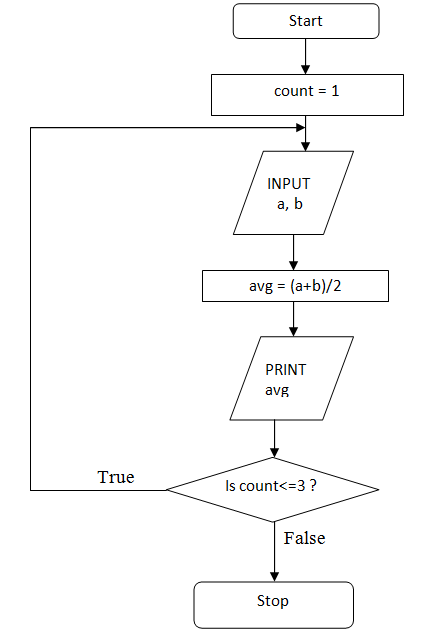C Language Code:

```#include <stdio.h>

int main()
{
int  a, b, avg, count ;

count =1;

do
{
printf("Enter values of a and b: ");
scanf("%d %d",&a,&b);
avg=(a+b)/2;
printf("Average = %d" , avg);
}while( count<=3 );

return 0;
}
```

Output

```    Enter the value of a and b: 2 4
Average = 3
Enter the value of a and b: 2 3
Average = 2
Enter the value of a and b: 2 6
Average = 4
```

What's New

Top Interview Coding Problems/Challenges!

Languages: » C » C++ » C++ STL » Java » Data Structure » C#.Net » Android » Kotlin » SQL
Web Technologies: » PHP » Python » JavaScript » CSS » Ajax » Node.js » Web programming/HTML
Solved programs: » C » C++ » DS » Java » C#
Aptitude que. & ans.: » C » C++ » Java » DBMS
Interview que. & ans.: » C » Embedded C » Java » SEO » HR
CS Subjects: » CS Basics » O.S. » Networks » DBMS » Embedded Systems » Cloud Computing
» Machine learning » CS Organizations » Linux » DOS
More: » Articles » Puzzles » News/Updates